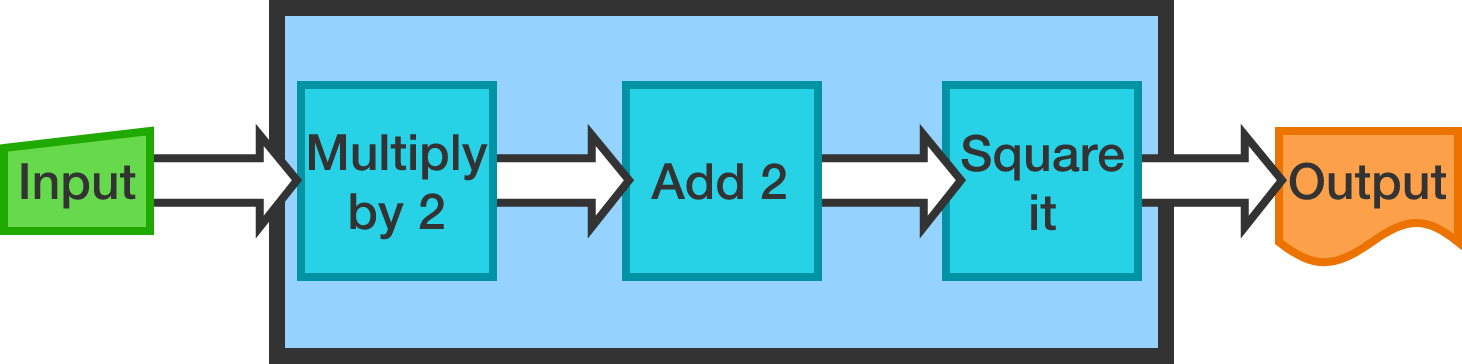# The Arithmetic Machine

Algebra Level 1

Alice and Bob have an arithmetic machine. The machine takes in a real number, does some processing and outputs a real number. Specifically, the machine works as follows:Now suppose the following:

• Alice entered a number into the machine and got back 100.
• Bob also entered a number and got back 100.

Does this mean that both Alice and Bob entered the same number?

×